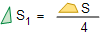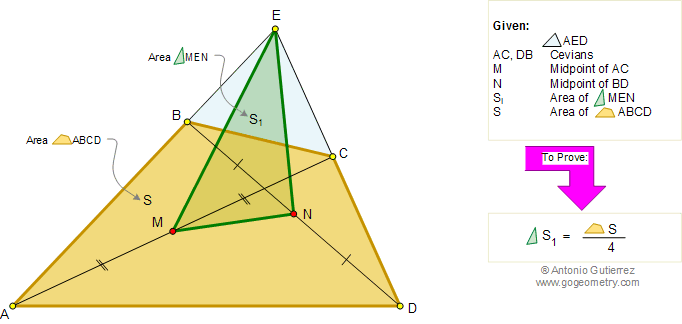# Geometry Problem 90. Area of Triangle and Quadrilateral, Midpoints of Diagonals, Median of a triangle. High School, College, Math Education

 In the figure below, given a triangle AED, M and N are the midpoints of cevians AC and DB respectively. If S1 and S are the areas of the triangle MEN and the quadrilateral ABCD respectively, prove that:.HINTS: 1. CEVIAN: A Cevian is a line segment which joins a vertex of a triangle with a point on the opposite side (or its extension). 2. See Problems 87, 89
 Home | Search | Geometry | Problems | All Problems | Open Problems | Visual Index | Art | 81-90 | Email | View or post a solution